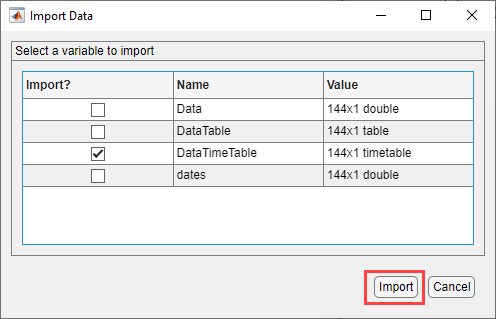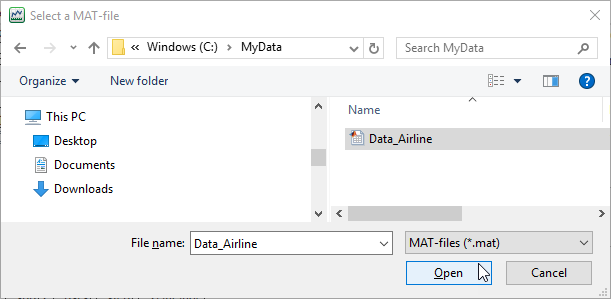## Import Time Series Data into Econometric Modeler App

These examples show how to import time series data into the Econometric Modeler app. Before you import the data, you must prepare the data at the MATLAB® command line (see Prepare Time Series Data for Econometric Modeler App).

### Import Data from MATLAB Workspace

This example shows how to import data from the MATLAB Workspace into Econometric Modeler. The data set `Data_Airline.mat` contains monthly counts of airline passengers.

At the command line, load the `Data_Airline.mat` data set.

`load Data_Airline`

At the command line, open the Econometric Modeler app.

`econometricModeler`

Alternatively, open the app from the apps gallery (see Econometric Modeler).

Import the MATLAB timetable containing the data:

1. On the Econometric Modeler tab, in the Import section, click.

2. In the Import Data dialog box, in the Import? column, select the check box for the `DataTable` variable.3. Click .

The variable `PSSG` appears in the Time Series pane, and its time series plot appears in the Time Series Plot(PSSG) figure window.The series exhibits a seasonal trend and serial correlation, but an exponential growth is not apparent. For an interactive analysis of serial correlation, see Detect Serial Correlation Using Econometric Modeler App.

### Import Data from MAT-File

This example shows how to import data from a MAT-file, stored on your machine, into Econometric Modeler. Suppose that the data set is named `Data_Airline.mat` and is stored in the `MyData` folder of your `C` drive.

At the command line, open the Econometric Modeler app.

`econometricModeler`

Alternatively, open the app from the apps gallery (see Econometric Modeler).

Import the MAT-file containing the data:

1. On the Econometric Modeler tab, in the Import section, click Import > Import From MAT-file.

2. In the Select a MAT-file window, browse to the `C:\MyData` folder. Select Data_Airline.mat, then click .3. In the Import Data dialog box, in the Import? column, select the check box for the `DataTable` variable.4. Click .

The variable `PSSG` appears in the Time Series pane, and its time series plot appears in the Time Series Plot(PSSG) figure window.